Vector Calculus - Mathematical Methods of Physics, UGC - NET Physics

# Vector Calculus - Mathematical Methods of Physics, UGC - NET Physics - Notes | Study Physics for IIT JAM, UGC - NET, CSIR NET - Physics

 1 Crore+ students have signed up on EduRev. Have you?

Dot Product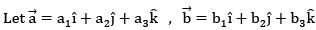are two constant vectors.

Then Dot Product of two vectors is given by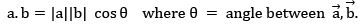Note: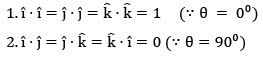3. Magnitude of Vector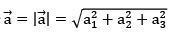4. Angle between two vectors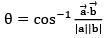5. Also,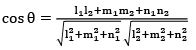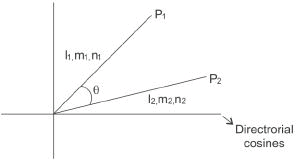6. If slopes are given and angle between two curves is θ then tan θ =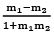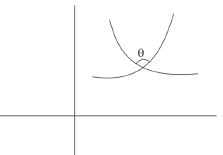7. If =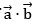= 0 ⇒ Vectors are orthogonal (θ = 90°)

8. If =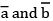= |a||b| ⇒ Vectors are parallel (θ = 0 )

Cross Product

If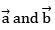are two vectors then then cross product between two vectors is given by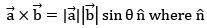= unit vector normal to bothNote: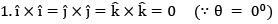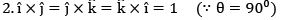3. Angle between two vectors is θ then sin θ =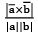4.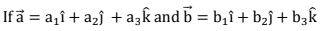then cross product between two vectors

can be calculated as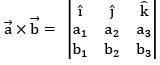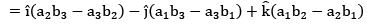5. Geometrically cross product gives the area of triangle

6. If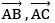are two sides of triangle then area of the triangle is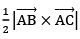Triple Product

1. Geometrically Triple Product gives the Volume of Tetrahedron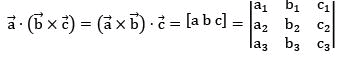2. If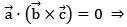Vector are coplanar vectors

Derivative of a Vector: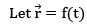be a position vector where t is a scalar variable.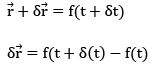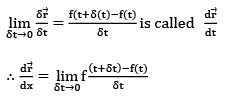Vector differentiation is nothing but ordinary differentiation but only difference is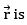position vector.

Formulae: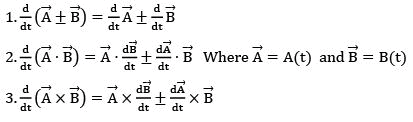Vector Operator (∇ - Del)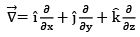is called vector operator

If ϕ (x, y, z) be a given scalar function then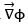is called gradient.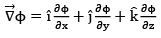Note:

1. Physically, gradient gives rate of change of ϕ w.r.t x, y, z separately.
2. Geometrically, it gives normal to the level surface.

Example 1: If ϕ = xyz then find the value of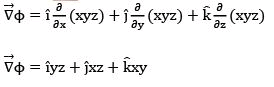Note: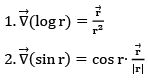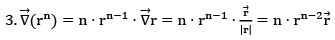4. Let ϕ(x,y,z) = c be given equation of the level surface then the outward unique normal vector is defined as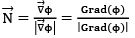Example 2:

Find the value of unit normal vector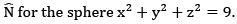Solution: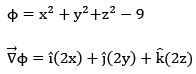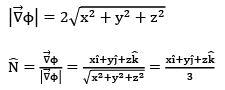Angle between Two Surfaces

Let ϕ1(x,y,z) = C, ϕ2(x,y,z) = C be given equations of two level surfaces and angle between these two surfaces are given as θ then cos θ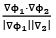Note:

The angle between two surfaces is nothing but the angle between their normal.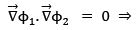then they are said to be orthogonal surfaces

Example 3:

The angle between the two surfaces x2 + y2+ z2 = 9 and z = x2 + y2 − 3 at the point (2, −1, 2) is

Solution: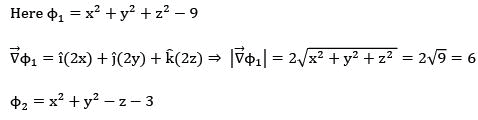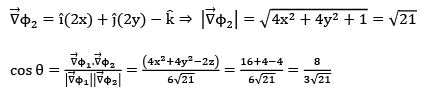Directional Derivatives of a Scalar Function

The directional derivative of a scalar function ϕ (x, y, z) in the direction of a vector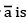given as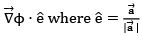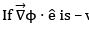ve then it is in the opposite direction.

Example 4:

The Directional derivative of f(x, y, z) = x2yz + 4xz2 at (1, −2, −1)along (2i – j − 2k) is
Solution: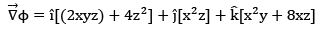Directional Derivative =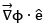At (1, −2, −1) we have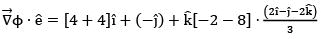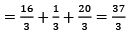Divergence of a Vector

If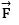is a vector point function then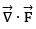is called Divergence ofWhere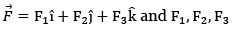are the functions of x, y, z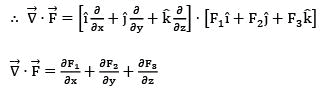Note:

1. Divergence of a vector is scalar.
2. Physically Divergence measures (outflow - inflow)
3. A vector whose divergence is zero then it is said to be divergence free vector (or) solenoid vector i.e. outflow = inflow = constant
4. Geometrically, Divergence gives the rate at which the fluid entering in a rectangular parallelepiped per unit volume at the point.

Curl of a Vector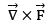is called the curl of a vector where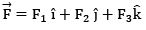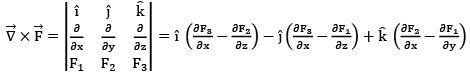Note:

1. If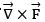= 0 then it is said to be irrational vector otherwise it is said to be rotational vector.

2. Physically Curl gives the angular Velocity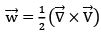3. Divergence of a curl of any vector is always zero.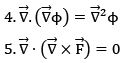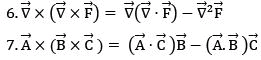is known as a vector triple product.

Example 5:

The values of a, b, c so that the vector,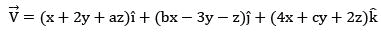is irrotational

Solution:

Given, that vector V is irrotational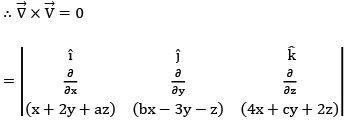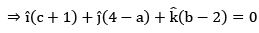⇒ c = −1, a = 4, b = 2

∴ a = 4, b = 2, c = −1

The document Vector Calculus - Mathematical Methods of Physics, UGC - NET Physics - Notes | Study Physics for IIT JAM, UGC - NET, CSIR NET - Physics is a part of the Physics Course Physics for IIT JAM, UGC - NET, CSIR NET.
All you need of Physics at this link: Physics

## Physics for IIT JAM, UGC - NET, CSIR NET

159 docs
 Use Code STAYHOME200 and get INR 200 additional OFF

## Physics for IIT JAM, UGC - NET, CSIR NET

159 docs

Track your progress, build streaks, highlight & save important lessons and more!

,

,

,

,

,

,

,

,

,

,

,

,

,

,

,

,

,

,

,

,

,

,

,

,

,

,

,

,

,

,

;